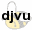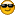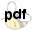###Author Topic: quantum level question  (Read 2526 times)

0 Members and 1 Guest are viewing this topic.

#### superman

• Guest##### quantum level question
« on: July 18, 2004, 05:04:00 AM »
just want to see if i'm understanding this.

2n^2, n=quantum level

iodine atom as an example

n1 has 2 electrons, n2 has 8, n3 has 18, n4 has 17 because the last level, n5 must contain 8 electrons

am i following this correctly?

#### b159510

• Guest##### Pauli or aufbau
« Reply #1 on: July 18, 2004, 08:45:00 AM »
Are you asking how to fill orbitals, or what the maximum number of electrons an energy level can have? 2n^2 is the maximum number of electrons that can be found in a given orbital/energy level, (restricted by the Pauli exclusion principle). But sublevels are often not filled, (see Hund's rule, etc). For iodine, n5 has 5 electrons, not 8. Even with the two electrons of the s orbital, the valence is only 7. It looks like your total is 53 though, which I think is correct. Interesting.

Actually n5 does have 7 electrons, not 5 like I said.
n5 includes all the valence-shell electrons.

#### superman

• Guest##### i'm reading Modern Inorganic Chemistry by C.
« Reply #2 on: July 19, 2004, 09:49:00 AM »
i'm reading Modern Inorganic Chemistry by C. Chambers and it appears that orbitals and quantum levels are two different things.    i think i have it figured out now though,   must have been tired when i was reading this

an orbital is charactorized as having a single energy level able to accomodate 2 electrons.

so n1 would contain 1 orbital, n2 has 4, n3=9, n4=9(this is the part that confuses me,   an orbital of n4 is missing an electron),    n5=4 (because the last quantum level must contain 8 electrons according to this text)

i just noticed this text was printed in 75,    is it obsolete?

#### b159510

• Guest##### Best of luck to you
« Reply #3 on: July 19, 2004, 07:28:00 PM »
Sorry. I'll have to defer to someone else to answer your question. I don't understand what you're asking, and I probably wouldn't do a good job of answering it anyway.

Wow. Ever since I got my masters in chemistry, I figured that I could always teach chemistry at the high school or junior college level if I needed a back-up job. I think I'll do students everywhere a favor and scratch teaching off my list of possible jobs.

#### jsorex

• Guest##### Yes orbitals are "groups" of ...
« Reply #4 on: July 19, 2004, 11:52:00 PM »
Yes orbitals are "groups" of electron states each characterized by a given value of the principal quantum number and the orbital quantum number. Thus the total number of states in an orbital with the given (orbital) value x, is 2(2X+1) (see Schrödinger equation)

#### lugh

• Guest##### Hybridization of Atomic Bonds
« Reply #5 on: July 20, 2004, 04:16:00 AM »
This article by Linus Pauling, who first theorized the hybridization of atomic orbitals, The Significance of Resonance to the Nature of the Chemical Bond and the Structure of Molecules; pp 1943-83 from Gilman's Organic Chemistry, An Advanced Treatise should answer your questions as well as many others#### superman

• Guest##### mmmmm, that's the stuff lugh :)
« Reply #6 on: July 20, 2004, 10:47:00 AM »
mmmmm,    that's the stuff lugh#### lugh

• Guest##### More Articles on Orbitals and Bonding
« Reply #7 on: October 01, 2004, 03:40:00 AM »
Some more articles on molecular orbitals and bondingLewis Structures of Boron Compounds Involving Multiple Bonds
JCE 72 6, 494-499 (1995)Non Koopmans' Molecules
JCE 72 6, 501-4 (1995)An Attention Getting Model for Aromatic Orbitals
JCE 72 6, 500 (1995)Bond Energies and Enthalpies
JCE 72 6, 497-99 (1995)#### claude

• Guest##### I think...
« Reply #8 on: October 03, 2004, 03:52:00 PM »
I think you are reffering to the orbitals, and the number of electrons that can be put in each of them. The orbitals are :

1s 2s 2p 3s 3p 4s 3d 4p 5s 4d 5p 6s 4f 5d 6p 7s 5f 6d

Orbitals s can contain 2 electrons, orbitals p can contains 6, the d 10, and f 14.
Have a look to the periodic table : the two firsts columns are orbitals s, the last six are p, the middle is d, with the introduction of the f at the element lanthane. There is a little exception, electrons of He, despite the fact that it is at the right, are in the 1s orbital.
H is 1s^1 for example, and K is 1s^2 2s^2 2p^6 3s^2 3p^6 4s^1. For iodine, you will have :
1s^2 2s^2 2p^6 3s^2 3p^6 4s^2 3d^10 4p^6 5s^2 4d^10 5p^5

#### indole_amine

• Guest##### there are different types of quantum numbers!
« Reply #9 on: October 22, 2004, 01:25:00 AM »
Superman and b159510:

Jsorex was the one with the "correctest" explanation so far, I think - although he did not exactly hit the nail on its head - but at least he used the hammer "quantum number = sum of electron states" - the right kind of tool...Quantum numbers distinguish between particles and their states (note the plural!). Besides quantum numbers depicting common quantities like spin s or charge Q, there are also a few others:

Angular track momentum L
Isospin I
Charm c
Overall spin J
z-component of the isospin I3
Strangeness S
z-component of the spin Sz
baryon number B
hyper charge H
lepton number L

According to Pauli, the electronic states of a single atom must only be occupied in a way that allows for not more than 2 electrons to correlate in all quantum numbers. This implicates that for example with helium, one electron has to have an up-spin (s=+1/2) while the other must have a down-spin (s=-1/2).

Particles (read: electrons too) can only take on an angular momentum of n/2 * h_ = h/2pi (h = Planck quantum effect number (?translation?)).
So the smallest spin unit is x*h_/2 (with x=1), and the number representing the factor x is called the quantum number in this case.

To indicate the spin of a particle, it therefore suffices to give the factor with which h_ has to be multiplied to give the spin. This number is also called the quantum spin number s.

There are particles with fermionic spin (numbered h/2, 3h/2, 5h/2 etc.) and bosonic spin (numbered 0, h, 2h, 3h etc.).

Other quantum numbers are numbered like this, for example: charge Q is either a multiple of the elemental charge e or 1/3rd of it, the maximum value of the isospin I is n/2-1/2 with n being the number of particles forming a theoretical nucleon (i.e. neutron and proton, where n=2); the hyper charge H is calculated from charge Q and isospin I3 according to the formula Y = 2(Q - I3) (i.e. proton and neutron have the same hyper charge!) - strangeness S and charm C are quantum numbers solely used for differentiating between quarks...

Hope this helps.

(Superman: you maybe could've used GOOGLE to read about it: search for "quantum number pauli principle"...
b159510: of course I'm a bit disappointed, because of your nickname, and, well - you know what I mean...)

...and to complete your confusion: the quantum number of a complex particle is the sum of its modules quantum numbers.
(And of course quantum numbers just depict particle states that can only be described in portions or quantums - not necessarily just their detention probability/orbital shape, but also track angular momentum I, being quantified into the quantum numbers 1,2,3,4,5 etc. (being correspondant to S,P,D,F,G,H etc)...)

indole_amine

#### indole_amine

• Guest##### refs.. (ebook: Modern Inorganic Chemistry)
« Reply #10 on: October 22, 2004, 04:01:00 AM »
Maybe someone would like to, but cannot participate in the discussion - but needs just the right literature on quantum mechanics...Modern Inorganic Chemistry (an intermediate text)
(C. Chambers and A.K. Holliday)
(Butterworth & Co. (Publishers) Ltd., London 1975)(pages 1-230; 9.1MB)[p. 231-459; 9.5MB)

(I also have "Frederic George Mann: Practical Organic Chemistry" as PDF, but I'm not sure whether I should upload it(?), since Polverone posted about having it on his personal website some time ago , and he linked to it, too AFAIK... so maybe uploading it isn't really necessary(?) If you think I'm horribly wrong about that, feel free to tell me, and I'll start cutting the PDF into uploadable pieces right away...)

indole_amine

#### superman

• Guest##### yup, you hit it! for a while i was spending...
« Reply #11 on: October 29, 2004, 04:34:00 AM »
yup,    you hit it!

for a while i was spending even more time on this stuff than chemistry until i got hung up on this, but i think you just kicked rekindled my interest!

much appreciated

#### somuchclass

• Guest##### Originally posted by superman: "Am I...
« Reply #12 on: October 29, 2004, 02:24:00 PM »
Originally posted by superman:

"Am I getting this correctly?"

The answer is sort of.  You got the number of electrons in the first couple of quantum levels right.  Namely, s has 2, then the s along with the 6 p's makes 8, then the 2 electrons associated with the s level along with the 6 of the p's and the 10 of the d's makes 18, but after that you will get 32 as your answer for the total number of electrons in the atom completing the f series (which atom that is I don't know but could easily tell you by glancing at the periodic table; feel free to help yourself).  And the atom denoted as having the first filled g level, if it were to occur naturally, would have a total of 50 electrons.

The mathematical algorithm being followed here is that, every time you move up a letter, the maximum number of electrons in the next quantum letter's completed shell will always be 4 more electrons than in the quantum energy level letter below it.  Namely, s is 2, p is 6, d is 10, f is 14 and so on until the atoms get so big and unstable as to become radioactive.

Now, the total number of electrons in an atom with a completed quantum level designation (those on the ends of the period table's rows) will simply be the number of electrons contained in that letter's last completed orbitals (e.g., 2 for s's or 6 for p's) using the Aufbau principle plus all the electrons in all of the lower energy shells summed together.  I hope this makes sense to you, if not then please allow me to elaborate further and you may eventually understand.

For example, I know the next number in the series that you stated after 18 is going to be 32 based on the following analysis.  That atom (whatever it may be) is going to have 2 electrons in its inner, filled s orbital, 6 electrons from the three inner p orbitals, 10 electrons from its five inner d orbitals, and 14 electrons from its outer, filled f orbitals.  Since 2 + 6 + 10 + 14 = 32 then that is the correct answer to your question.  Keep in mind that an orbital is simply defined as being the 3 dimensional shape that the electrons which compose it have a 90% chance of occupying at any given moment.

Interestingly, if you really want to think about this some more, Huckel's empirically derived rule for whether a conjugated, organic molecule is aromatic, anti-aromatic or non-aromatic is based on the general formula H(n) = 4n + 2, where n is the number of delocalized p orbital overlapping electrons (which form pi bonds with each other due to their partial overlap amongst one another) in a cyclic, all sp2 (120 degree bond angles) hybridized carbon atoms containing molecule.

This equation corresponds exactly (if you arbitarily associate s with n = 0, p with n = 1, d with n = 2 and so forth) with the inorganic quantum mechanical discussion we've been having based on the original question that you posed at the beginning of this thread, and this correlation  suggests that a benzene ring may actually be most correctly viewed as one large "supra" atom composed of 6 smaller C atoms and 6 hydrogen atoms attached to systematically to those carbons whose inner orbitals have hybridized with each other to create a structure which cannot be represented by the traditional, quantized Kekule system of drawing molecular structures by connecting all their atoms with lines to represent bonds and making sure that each atom has its characteristic number of bonds needed to satisfy its valence demands.

That is, the interatomical interactions between the molecules which make up benzene behave in much the same way that an f orbital spontaneously forms, based on myriad physical interactions between its nucleus of protons and neutrons and its electron cloud orbitals surround that nucleus--shielding is one example of such a force, as soon as the inner s, p and d orbitals have become filled.  The net result, in each case, is a molecular entity that can only be described as a permutated sum of its original parts.  The difference in energy between the sum of the bond energies of benzene versus its actual energy content as measured thermodynamically by burning a given amount in a bomb under high oxygen pressure and then measuring the rise in temperature which results using the equation q = mc(delta)T, where q is heat, m is the mass burned, c is the heat capacity of the substance and delta T is the change of temperature that results.  The difference between the predicted energy value of a given quantity of benzene based on the sum of its atomic constituents versus the actual measured energy value of the same amount of benzene is called the molecule's resonance energy.  Resonance energy is a characteristic of aromaticity.  The term which is analogues to resonance energy within the world of inorganic chemistry is dubbed mass defect and, not surprisingly, describes the difference in energy of an atom in reality compared with what the energy of that atom would be predicted to be based on all its constituent quarks and what not.

This last major statement can be visualized by making the analogy of a child who, taking some blocks and instead of using the blocks as is to construct a toy fortress, gets creative on his parents' asses and actually both distorts the shapes of the blocks and then builds some kind of new creation of lesser energy than the sum of the energies of all of their bonds.  The individual building blocks have both been summed together and themselves intrinsically changed in the process.

In the case of benzene, the value of n using Huckel's rule is 4(1) + 2 = 6, so there n is obviously one.  If n is decreased to zero, then you have a situation not like that observed by the likewise highly stable (high stability is a characteristic of aromaticity) lone pairs of electrons found on nitrogen or oxygen atoms, for example.

But what, you may ask, if n is increased by a factor of one to that where n = 2, then you get 4(2) + 2 = 10?  It is tantalizing tempting to declare the benzodiazepine nucleus, which is a 7 membered cyclic organic entity with 10 pi electrons (keep in mind that the lone pairs of N, O or S can be counted as "pi electrons" if they are geometrically aranged in space correctly with respect to the molecule's other pi electrons), such an example; alas, there is one insulating sp3 hybridizing carbon among the ring system, so a more complicated theoretical model which takes that anomaly into account may be called for, and no, this time I'm really not babbling (or maybe I am).  Either way, it's only logical.  Hope that helps.